GitHub

# Displaying data in H2O Wave

Author: Georgia Atkinson

Published: June 16, 2022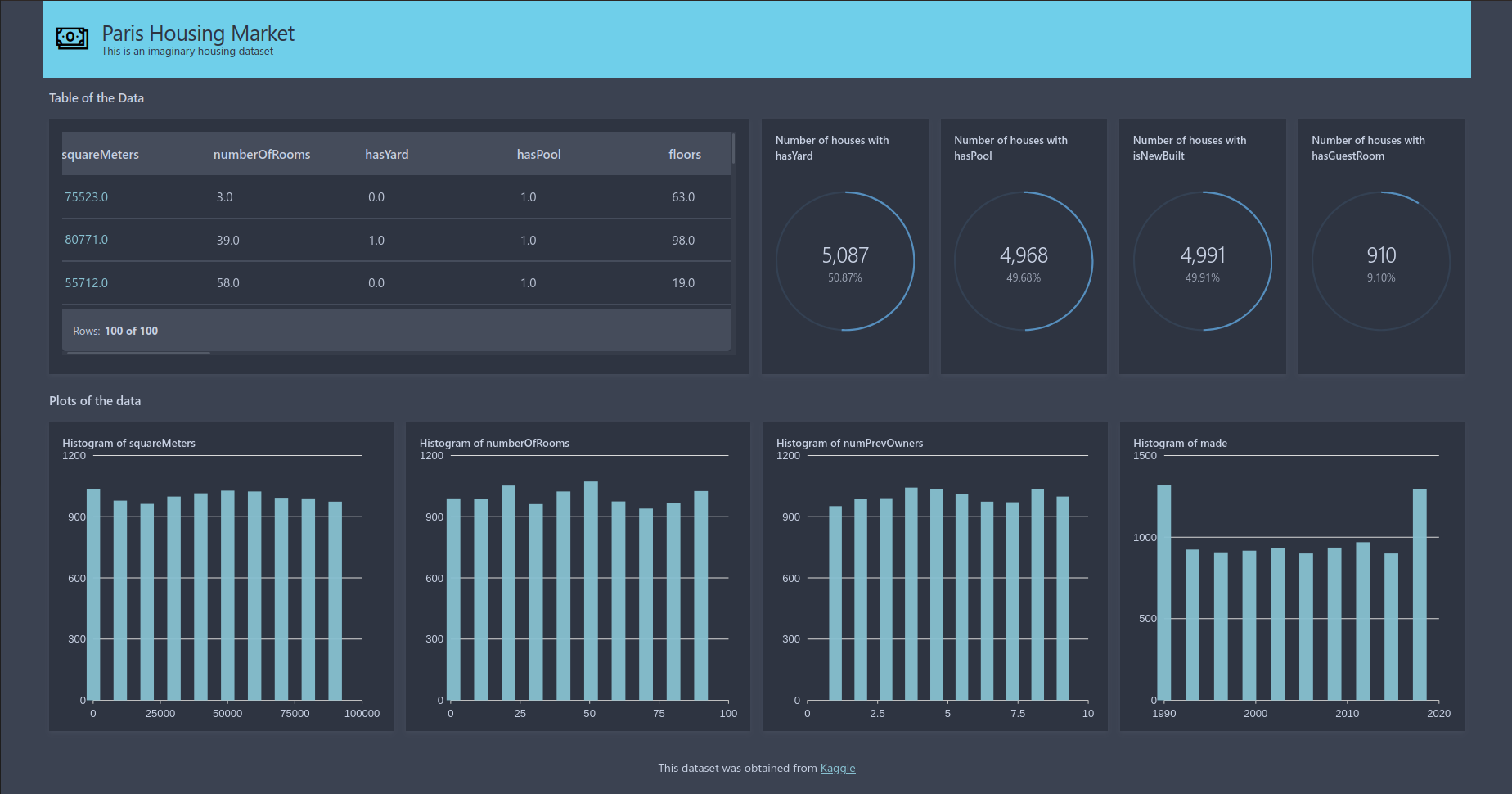If you’re used to coding in Python or R and want to write a web application quickly you can avoid having to learn HTML, JavaScript, etc, by using H2O Wave to write your web application with Python or R. In this post we will go through an example of how to build a simple app to display data in various forms including plots, tables and graphics using Python.

Before continuing with this tutorial if you have no experience with H2O Wave I would strongly suggest going through their introductory tutorials and installation guide first before you proceed. The introductory tutorials will talk you through how to set up basic apps, starting with the classic ‘Hello World’ example that we all know and love and also show you how to install H2O Wave.

To start, I would suggest creating a Python script called `app.py`, this is where we will write all of our code. To see how the app develops throughout this tutorial, from the terminal we can run:

``````wave run app.py
``````

If this does not work, make sure you have `h2o_wave` installed in the python environment that you are using.

## The dataset

For this tutorial we will be using an imaginary housing dataset which you can download from Kaggle. It gives a variety of information on housing stock (e.g. number of rooms, square footage, etc…) and their pricing. We will make a simple app to display some of these data.

Data comes in all shapes and sizes. It can often be difficult to know where to start. Whatever your problem, Jumping Rivers can help.

## The basic setup

Whenever I start writing a Wave app I always start with the basic setup which we will use for our web application.

``````from h2o_wave import Q, ui, app, main

@app('/displayData')

async def server(q: Q):

apply_layout(q)
show_homepage(q)

await q.page.save()

def show_homepage(q: Q):
pass

def apply_layout(q: Q):
pass
``````

The first thing we do is import `h2o_wave` and define where to listen to the user interface with `@app()` (in our code that will be `localhost:10101/displayData`). If we now save `app.py` and visit `localhost:10101/displayData` in a browser we should see a blank web page. If you keep this browser open, every time we save `app.py` the browser will update to show the latest additions to our web application.

We then define a function `server()` which will be run every time a user interfaces with `localhost:10101/displayData`. Inside `server` I always call two functions:

• `apply_layout()` where we define the layout of the app.
• `show_homepage()` which will be the default loading page for the app.

We’ll start by creating a basic homepage layout that will only include a header and a footer for now, but we will add to this function as we go. We will then add pages to `show_homepage()` to display the header and footer.

``````def apply_layout(q:Q):
q.page['meta'] = ui.meta_card(
box='',
theme='nord',
layouts=[
ui.layout(
breakpoint='xl',
width='1600px',
zones=[
ui.zone('footer')
]
)
]
)

def show_homepage(q: Q):
width='100%',
height='86px'),
icon='Money',
icon_color='Black',
title='Paris Housing Market',
subtitle='This is an imaginary housing dataset')

q.page['footer'] = ui.footer_card(
box='footer',
caption='This dataset was obtained from [Kaggle](https://www.kaggle.com/datasets/mssmartypants/paris-housing-price-prediction)')
``````

In `apply_layout()` we add a page, `meta`, to create a `meta_card` object. We specify a theme for our app using the `theme` argument and also define a web app layout using the `layout` argument. The most important part of `apply_layout()` is contained within `ui.layout` for our `layout` argument. This is where we specify different zones by using `ui.zone()`. We define a `header` zone for the header and a `footer` zone for the footer. Note that a zone can be made up of multiple zones which we’ll see later on.

In `show_homepage()` we add two cards:

• `header`. This uses the `ui.header_card` to define the header for our app. The `icon` argument references UI Fabric icons.
• `footer`. This uses the `ui.footer_card` to define the footer for our app. The `caption` argument takes string input but recognises markdown, so you can easily add links like we have here.

Both cards have the argument `box`. We use `box` here to link the cards with our layout in `apply_layout()`. For example, we have a footer zone in our layout function labelled `footer`. Setting `box = 'footer'` inside `ui.footer_carder` informs the code to place the footer card where we have specified it to be inside `apply_layout()`. If we want to be more specific with the box location we can the function `ui.box` for the `box` argument, this is what we do inside `ui.header_card`. Here we have specified the width of the box using the `width` argument, and the height using the `height` argument. Pass `width = 100%` will make the box the width of the app.

If you save `app.py` you should see the web app update in your browser to have a header and footer, like in the image below.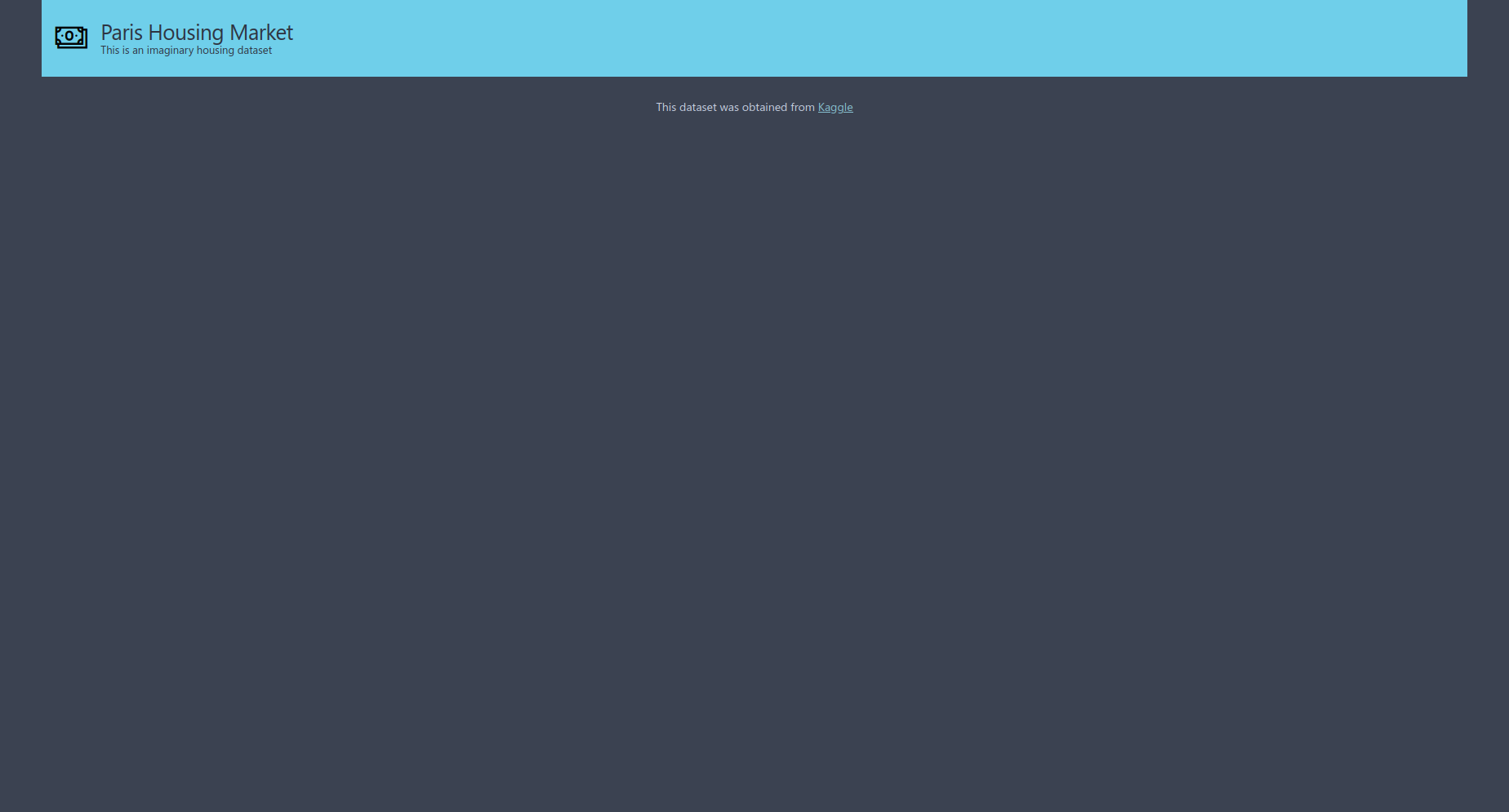The first thing we’ll add to our app is a table to view the raw data. To start we’ll need to read in our data with `pandas` and then we can create a table with `ui.table()`, which we’ll put into a function called `show_table()`. Note that we will only load the first 100 rows of the table otherwise the app is slow to load.

``````import pandas as pd

def make_table(df):
return ui.table(name='table',
height = '250px',
columns = [ui.table_column(name=x, label=x) for x in df.columns.tolist()],
rows = [ui.table_row(
name = str(i),
cells = list(map(str, df.values.tolist()[i]))
) for i in df.index[0:100]]
)

def show_table(q:Q, df):
q.page['section1'] = ui.section_card(box='section1',
title='Table of the data',
subtitle=''
)
q.page['table'] = ui.form_card(box=ui.box('top', width = '50%'),
items = [make_table(df)]
)
``````

Sadly, there is no seemingly simple way to display a `pandas.DataFrame` with H2O Wave so we have to be a little cunning with how we feed our data frame into `ui.table()`. If you look at the documentation for `ui.table()` it expects arguments `columns` and `rows` with type `List[TableColumn]` and `List[TableRow]` respectively, so we cannot simply feed the columns and rows of our data frame to `columns` and `rows`. Instead, what we can do is use list comprehensions to define the columns and rows which is what we do in `make_table()` above. Defining the columns is pretty straight forward, we use `ui.table_column()` to define each column and pass in the column name from `df.columns.tolist()`. Defining the rows is a little more complicated. We can use the same trick as we did for the columns by using `df.values.tolist()` to extract the rows, however the arguments in `ui.table_row()` expect strings, so we need to convert the values passed to `name` and `cells`. For the list comprehension we can simply iterate over an array with the row indices and convert the name of each row to a string using `str()` which we pass to `name`. For the `cells` we need to map the output from `df.values.tolist()[i]` to a string and then set this as a list.

Now that we’ve made our table with `make_table()` we can make a function `show_table()` to display it in our app. We can use a section card to give a title above the table with `ui.section_card()` and add the table by passing it to the `item` argument inside a form card. We specify where the card will go by using the `box` argument. Here we are using `ui.box()` to specify which zone our table is in with `'top'` and use the `width` argument to specify the desired space for the table. We need to then add these extra zones to `apply_layout()`.

``````def apply_layout(q: Q):
q.page['meta'] = ui.meta_card(box='', theme='nord', layouts=[
ui.layout(
breakpoint='xl',
width='1600px',
zones=[
ui.zone('content', direction=ui.ZoneDirection.COLUMN,
zones=[
ui.zone('section1'), ##NEWLINE
ui.zone('top', size='310px', direction = ui.ZoneDirection.ROW), ##NEWLINE
]),
ui.zone('footer')
]),
])
``````

Notice that we specify zones within zones here for the main body of our app. We specify `'content'` and use the `direction` argument which is useful to define the layout direction for the app. We also use the `direction` argument in our `top` zone. This instructs our app to only fill up 50% of the `top` zone. We also use the `size` argument to specify the size of the zone, which for us is the height of the zone. Try changing this argument and you will see it’s effect. We’ll fill in the other 50% of the zone with some stats cards which we’ll walk through below.

Finally, to get the table to display in our app we need to call the function inside `server`().

``````async def sever(q: Q):

apply_layout(q)
show_homepage(q)
show_table(q, df)

await q.page.save()
``````

Now that we’ve updated `server()`, save the code and your browser should update and look very similar to this image.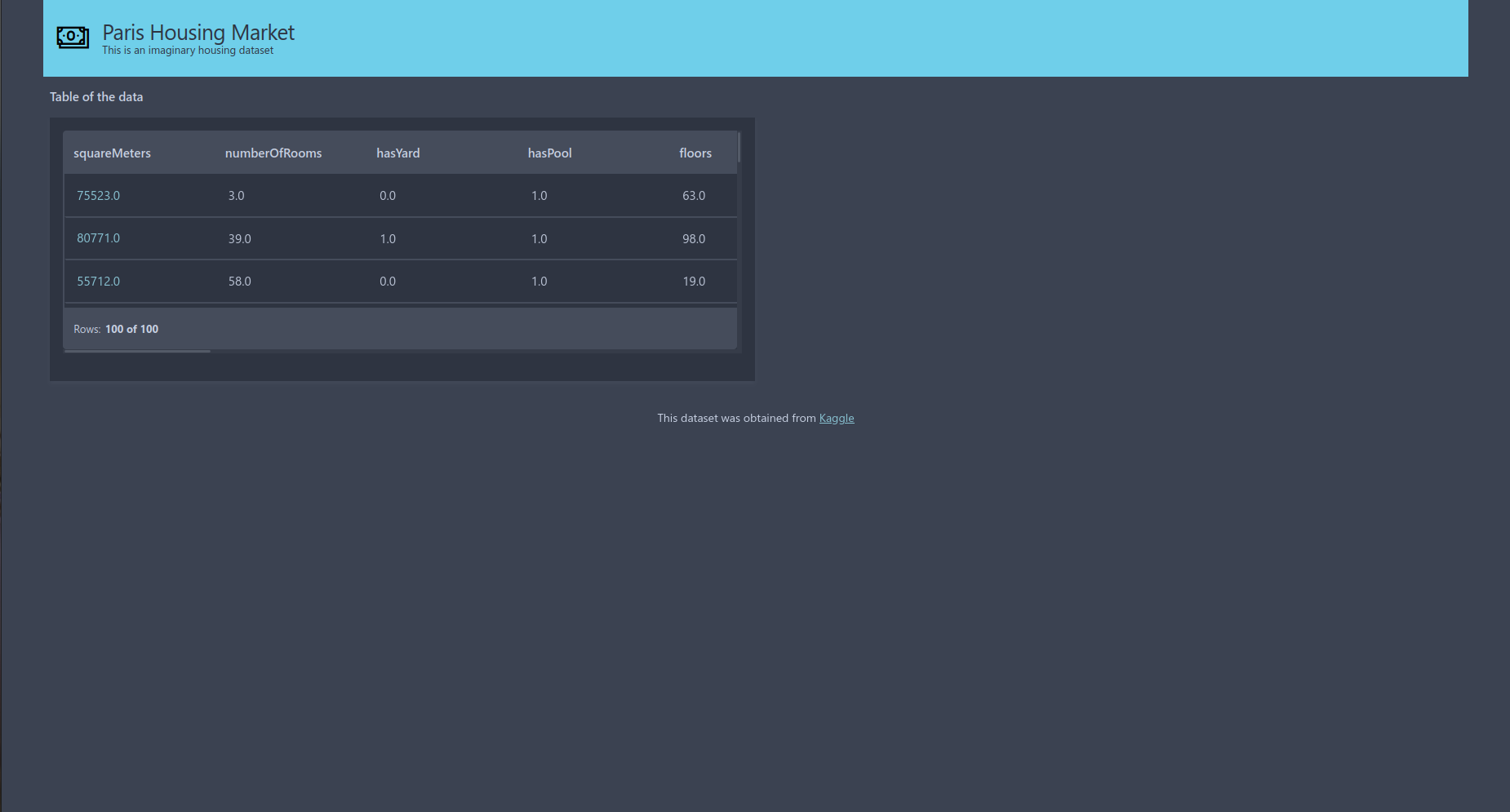Next we’ll add stats cards to our app to fill the space next to our table. Stats cards can be used in a variety of ways to display simple statistics. We are going to use them to display some binary data in our dataset, such as `'hasYard'`, by displaying the total number of attributes that are `1` (i.e. for `hasYard` the number of houses that have a yard).

``````def make_stats_card_data(q:Q, column):
value_counts = column.value_counts()
total = value_counts.sum()
return value_counts, total

def show_stats_card(q:Q, column, index):
value_count, total = make_stats_card_data(q, column)
percentage = value_count/total

q.page['stat'+str(index)] = ui.tall_gauge_stat_card(
box = ui.box('top', width = '11.75%'),
title = 'Number of houses with ' + column.name,
value = '={{intl one}}',
aux_value='={{intl perc style="percent" minimum_fraction_digits=2 maximum_fraction_digits=2 }}',
progress = percentage,
data = dict(one = int(value_count), perc = float(percentage)),
plot_color = '\$blue'
)

def show_multiple_stats_cards(q:Q, df, index):
for i in index:
column_name = df.columns.tolist()[i]
column = df[column_name]
show_stats_card(q, column, i)
``````

To start we need to transform the data that is passed to our stats card using `make_stats_card_data()` so it is in a suitable format. This counts the number of zeros and ones and sums the results to give the total. This is then used in `show_stats_card()`. Here we use a `tall_gauge_stat_card()` and using the same trick as before use `ui.box()` to define how much space our card takes up. Now to pass values to `value` and `aux_value` we need to pass information to our `data` augment. The simplest way to do this is by using a dictionary. We can then use the keys in our `value` and `aux_value` arguments. When looking at the documentation for a `tall_gauge_stat_card` we can see that the arguments just mentioned expect strings as their input. The standard way to pass arguments to these are by using `={{}}` notation and then define the type of the data. We then give the keys from our dictionary and any other commands, such as the style to display the value and the number of decimal points to display (the values in the middle of the stats cards example image below). The `progress` argument is used in the circle around the data (the light blue colour in the example image below) and should be between zero and one. Finally, you can pass the colour of the circle using `plot_color`, but your argument must be of the form `\$<COLOUR>`.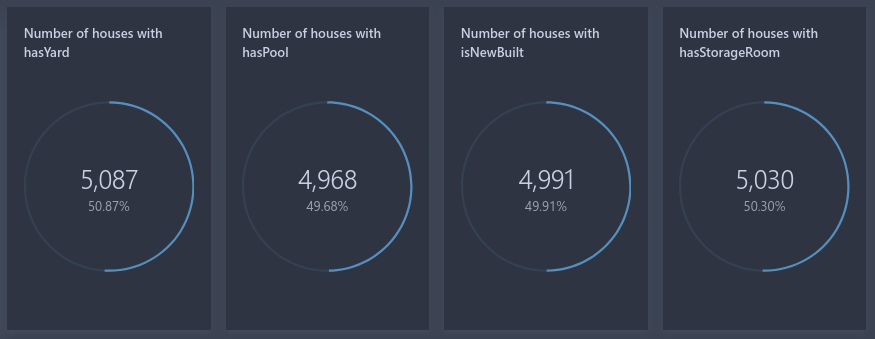Notice in `show_stats_card()` how we set up our page. We give a base name, `'stats'`, and then add an addition string which can be changed to allow the same piece of code to produce multiple stats cards. This is what we do in `show_multiple_stats_cards`, we simply loop over different indices which related to the columns so we make multiple cards. If we didn’t do this and kept all cards labeled `'stats'` only one stats card would appear in our app.

Like the table we created above, the final step is to add `show_multiple_stats_cards()` to `server()` and pass in columns that we want to display as stats cards. Here we have passed in indices of binary columns.

``````async def sever(q: Q):

apply_layout(q)
show_homepage(q)
show_table(q, df)
show_multiple_stats_cards(q, df, index = [2,3,9,14])

await q.page.save()
``````

After updating `server()` and saving your code you should see something very similar to this image.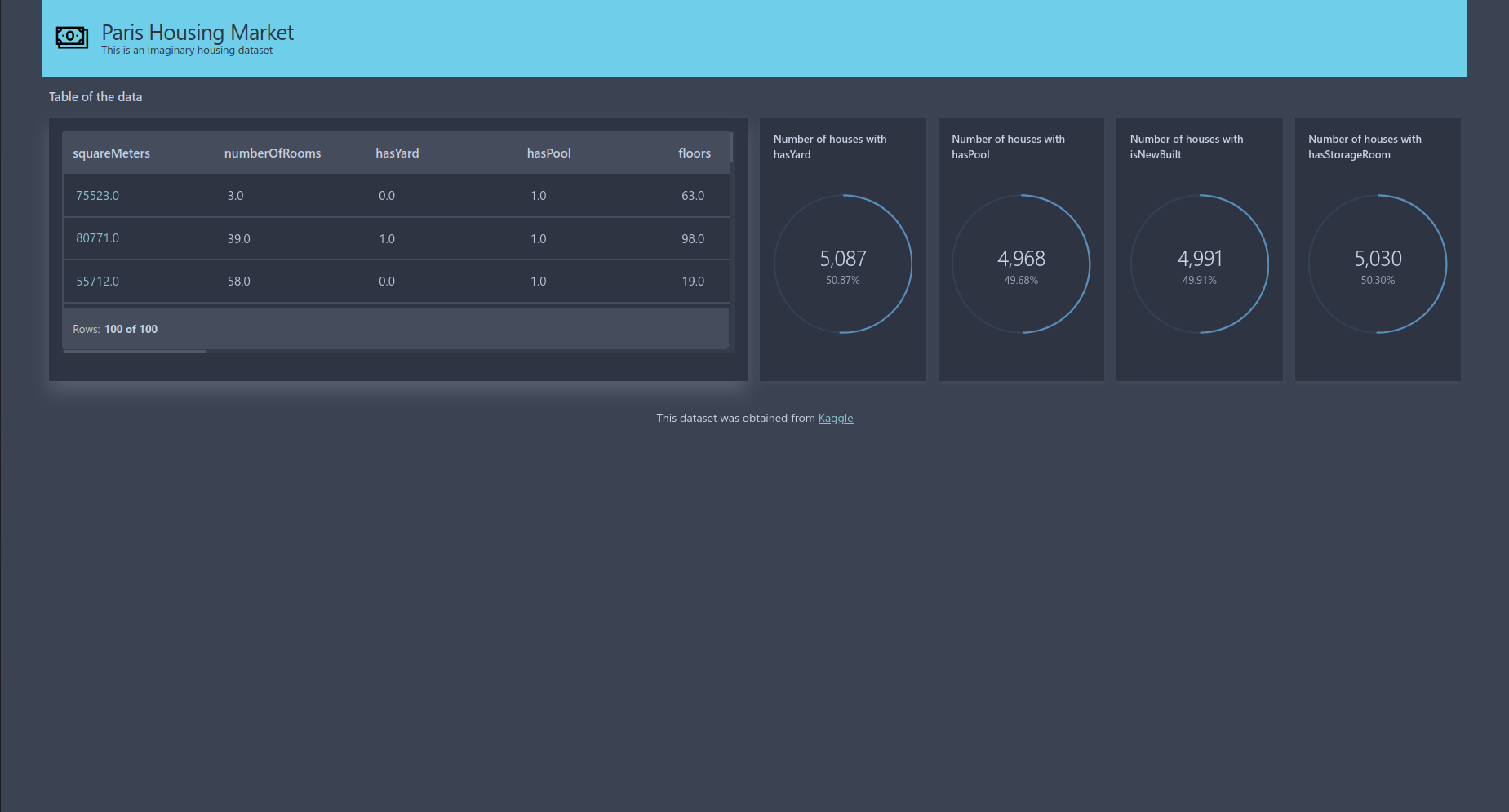The final items we will add to our app are some histogram plots. To add a histogram to our Wave app we first need to transform the data into bins and counts which we can then pass into a plot card, `ui.plot_card()`.

We can transform our data pretty easily thanks to `numpy`. We simply need to pass our data to the `numpy.histogram()` function and reshape it so it is ready for the plot card. This is what we have done in our `made_histogram_data()` function in the code below.

``````def make_histogram_data(values):
count, division = np.histogram(values)
return [(x, y) for x, y in zip(division.tolist(), count.tolist())]

def show_histogram(q:Q, values, variable_name, index):
q.page['feat'+variable_name] = ui.plot_card(
box = ui.box('bottom', width = '25%'),
title = 'Histogram of ' + variable_name,
data = data(
fields = ['division', 'count'],
rows = make_histogram_data(values),
),
plot = ui.plot([ui.mark(type='interval',
x='=division', y='=count',
y_min=0,
x_title=variable_name, y_title='Count')]
)
)

def show_histograms(q:Q, df, index):
q.page['section2'] = ui.section_card(box='section2',
title='Plots of the data',
subtitle='')

column_names = df.columns.tolist()[index]
for name in colum_names:
values = df[name]
show_histogram(q, values, name)
``````

The next step is to make a histogram plot, which we will define inside `show_histogram()`. We can use `ui.plot_card()` to create a histogram. Using the same trick as we have previously, we use `ui.box()` to define the zone to display our histogram and the width of the card. We use the `data` argument to pass in the output from `make_histogram_data()` with the `data()` function. Inside `data()`, we use the `fields` argument to name the columns of our data, and `make_histogram_data()` to define the `rows` of the data, where each row is `[division, count]`. Finally, we use the `plot` argument to specify our plot using `ui.plot()`. When looking at the documentation for `ui.plot()` we can see it expects one argument, `mark`, which expects a list object with class `Mark`. To do this we use `ui.mark()`. Inside `ui.mark()` we define the plot type, what variable to use, along with various other options you have in a typical plotting function. For us to produce a histogram we must pass `type='interval'`, and give `division` and `count` defined in `data()` to the `x` and `y` arguments. Note that we need to pass `x='=X'`, instead of `x=X` or `x='X'`. To tidy the plot up we also pass `ymin=0` to stop the histogram producing a negative y axis and also pass in some labels for the x and y axes with `x_title` and `y_title`.

To produce multiple histograms we use the same trick that we used when creating the stats cards by letting the page name vary in `show_histogram()`. This means when we create `show_histograms()` all we need to do is simply iterate `show_histogram()` over different column names with the relevant data and we will produce multiple histograms on our Wave app. We also add another section header into `show_histograms()` to give a heading to our histograms.

Next we need to update `apply_layout()` to define where the plot cards and section card will be placed. Similar to the `'top'` zone, we again use the `direction` argument to specify the layout direction to be a row. i.e. we want to space the cards horizontally. We simply need to add two extra zones within our content zone labelled `section2` and `bottom`.

``````def apply_layout(q: Q):
q.page['meta'] = ui.meta_card(box='', theme='nord', layouts=[
ui.layout(
breakpoint='xl',
width='1600px',
zones=[
ui.zone('body', direction=ui.ZoneDirection.ROW, zones=[
ui.zone('content', direction=ui.ZoneDirection.COLUMN, zones=[
ui.zone('section1'),
ui.zone('top', size='300px', direction = ui.ZoneDirection.ROW),
ui.zone('section2'),
ui.zone('bottom', direction=ui.ZoneDirection.ROW),
]),
]),
ui.zone('footer')
]),
])
``````

Then finally, we add `show_histograms()` to `server()` so the histograms will appear in the browser.

``````async def sever(q: Q):

apply_layout(q)
show_homepage(q)
show_table(q, df)
show_multiple_stats_cards(q, df, index = [2,3,9,14])
show_histograms(q, df, index = [0, 1, 7, 8])

await q.page.save()
``````

Now, you should see something very similar to the picture below in your browser.## The final code

Finally, here is the code in full that we have just produced above, hopefully your code should look very similar!

``````from h2o_wave import Q, ui, app, main, data
import numpy as np
import pandas as pd

values = df.values.tolist()
variable_names = df.columns.tolist()

@app('/displayData')

async def sever(q: Q):

apply_layout(q)
show_homepage(q)
show_table(q, df)
show_multiple_stats_cards(q, df, index = [2,3,9,14])
show_histograms(q, df, index = [0, 1, 7, 8])

await q.page.save()

def show_homepage(q:Q):
icon='Money',
icon_color='Black',
title='Paris Housing Market',
subtitle='This is an imaginary housing dataset')

q.page['footer'] = ui.footer_card(
box='footer',
caption='This dataset was obtained from [Kaggle](https://www.kaggle.com/datasets/mssmartypants/paris-housing-price-prediction)')

def apply_layout(q: Q):
q.page['meta'] = ui.meta_card(box='', theme='nord', layouts=[
ui.layout(
breakpoint='xl',
width='1600px',
zones=[
ui.zone('body', direction=ui.ZoneDirection.ROW, zones=[
ui.zone('content', direction=ui.ZoneDirection.COLUMN, zones=[
ui.zone('section1'),
ui.zone('top', size='300px', direction = ui.ZoneDirection.ROW),
ui.zone('section2'),
ui.zone('bottom', direction=ui.ZoneDirection.ROW),
]),
]),
ui.zone('footer')
]),
])

### Making table
def make_table(df):
return ui.table(name='table',
height = '250px',
columns = [ui.table_column(name=x, label=x) for x in df.columns.tolist()],
rows = [ui.table_row(name = str(i), cells = list(map(str, df.values.tolist()[i]))) for i in df.index[0:100]]
)

def show_table(q:Q, df):
q.page['section1'] = ui.section_card(box='section1',
title='Table of the Data',
subtitle='')
q.page['table'] = ui.form_card(box=ui.box('top', width = '49.5%'),
items = [make_table(df)],
)
## Making stats cards
def make_stats_card_data(q:Q, column):
value_counts = column.value_counts()
total = value_counts.sum()
return value_counts, total

def show_stats_card(q:Q, column, index):
value_count, total = make_stats_card_data(q, column)
percentage = value_count/total
q.page['stat'+str(index)] = ui.tall_gauge_stat_card(
box = ui.box('top', width = '11.75%'),
title = 'Number of houses with ' + column.name,
value = '={{intl one}}',
aux_value='={{intl perc style="percent" minimum_fraction_digits=2 maximum_fraction_digits=2 }}',
progress = percentage,
data = dict(one=int(value_count),perc=float(percentage)),
plot_color = '\$blue'
)

def show_multiple_stats_cards(q:Q, df, index):
for i in index:
column_name = df.columns.tolist()[i]
column = df[column_name]
show_stats_card(q, column, i)

### Making histograms
def make_histogram_data(values):
count, division = np.histogram(values)
return [(x, y) for x, y in zip(division.tolist(), count.tolist())]

def show_histogram(q:Q, values, variable_name, index):
q.page['feat'+str(index)] = ui.plot_card(
box = ui.box('bottom', width = '25%'),
title = 'Histogram of ' + variable_name,
data=data(
fields=['division', 'counts'],
rows=make_histogram_data(values),
pack=True,
),
plot=ui.plot([ui.mark(type='interval',
x='=division', y='=counts',
y_min=0,
x_title=variable_name, y_title='Count')]
)
)

def show_histograms(q:Q, df, index):
q.page['section2'] = ui.section_card(box='section2',
title='Plot of the data',
subtitle='')
for i in index:
column_name = df.columns.tolist()[i]
values = df[column_name]
show_histogram(q, values, column_name, i)
``````

## Next steps

Now that we’ve made our app why not go ahead and try to add other widgets to it or maybe change the layout or colour scheme? Take a look at the Wave documentation to see what else you could add! There are examples on how to use most widgets and step-by-step guides talking through various topics such as page layouts, uploading files and writing tests.

H2O Wave also have a GitHub repository with applications that they have made which you could try using and editing for your own use. If that isn’t enough links for you to look at, the Wave website also has a blog section where they announce any updates and share other useful information.

Finally, I always find it helpful to look through a few different examples of how to code something when I’m learning a new skill so why not take a look at this video tutorial made by H2O. The tutorial will show you how to create a simple app for displaying data a bit like what we have just gone through (but they make the app more interactive). Note that the tutorial was created in 2021 so it uses an older version of H2O Wave. It still works with the latest version of H2O Wave (which at the time of writing is 0.20), the only difference is how to run the app. In previous versions (below 0.20) we needed to start a wave server manually, but now we can simply run your wave app by `wave run <code_script>` and the wave server is started automatically.

Jumping Rivers are now an H2O.ai partner, so if you want any further information please feel free to contact us. You can also check out our courses on H2O Wave and H2O Driverless AI.

I hope you found this tutorial helpful. Have fun making your next H2O Wave app!